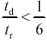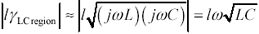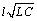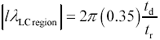# Pi-Model Operated in the LC Region

Suppose you intend to model a transmission line operating at a frequency at or above the LC region boundary. Constrain the line to a length l sufficiently short that the total line delay t d remains less than 1/6 of the signal risetime t r .

Equation D.12The next equations determine the effect of constraint [D.12] on the magnitude of the length-adjusted propagation coefficient l g . In the LC region the inductive reactance w L by definition exceeds R , so you may safely approximate the magnitude of the coefficient l g by omitting the R .

Equation D.13The midpoint of the spectrum associated with the rising and falling edges of the signal driving the line is related to the rise and fall time t r .

Equation D.14Evaluating [D.13] at w edge , and recognizing that for an LC-mode transmission line the effective line delay t d equals,

Equation D.15At a ratio of t d / t r = 1/6 the coefficient l l LCregion equals .366, at which value the pi-model error given by [D.11] ( assuming the ratios Z S / Z C and Z C / Z L to each be less than 3.8) works out to about 3%. At a ratio t d / t r = 1/3 the error soars to 25%. Above t d / t r = 1/2 the pi model loses all useful predictive power.High-Speed Signal Propagation[c] Advanced Black Magic
ISBN: 013084408X
EAN: N/A
Year: 2005
Pages: 163